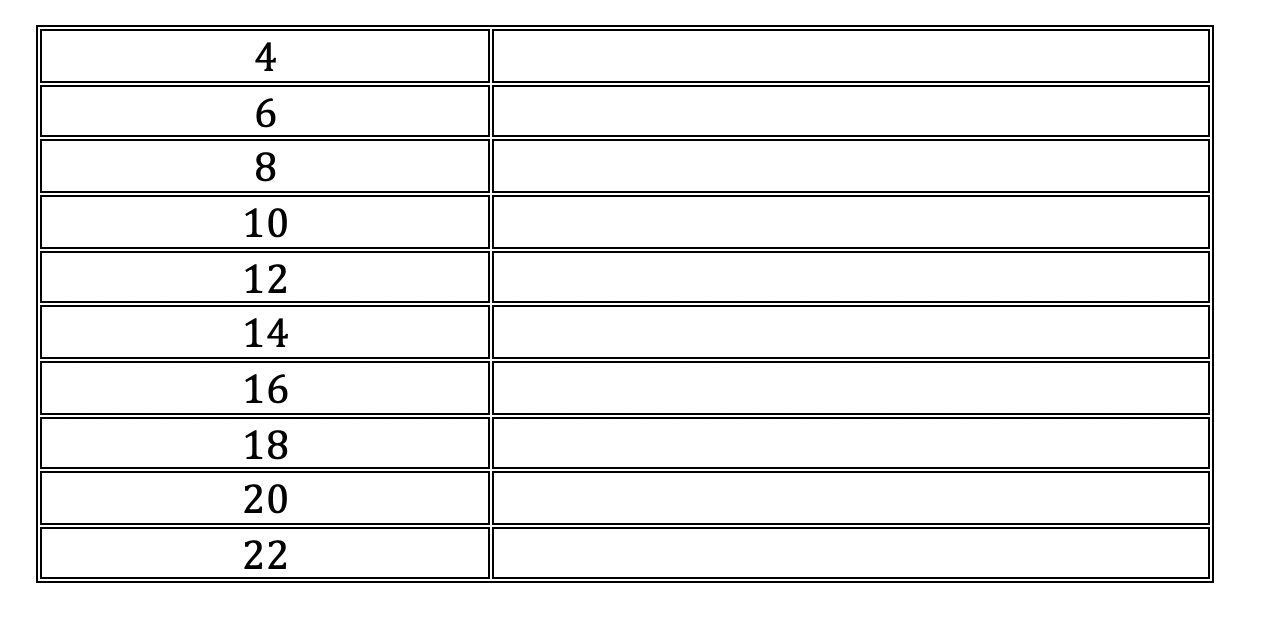# Week 11: A \$1 million maths problem (Goldbach)This week’s problem gives you a chance to become famous (and potentially rich)!  German mathematician Christian Goldbach (above) in 1742 proposed the following conjecture:

“Every even integer greater than 2 can be written as the sum of 2 prime numbers.”

Over 250 years later no-one has been able to prove it.  Anyone who can prove it get \$1 million and would enter the mathematical history books!  Can you find make a start by finding all the solutions to following?